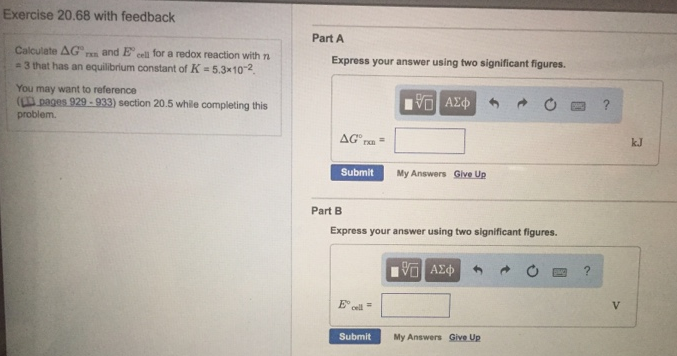# Problem: Calculate ΔG°rxn and E°cell for a redox reaction with n = 3 that has an equilibrium constant of K = 5.3 x 10-2. You may what to reference (Pages 929 - 933) section 20.5 while completing this problem. Part AExpress your answer using two significant figures. Part BExpress your answer using two significant figures.

###### FREE Expert Solution
90% (32 ratings)###### Problem Details

Calculate ΔG°rxn and E°cell for a redox reaction with n = 3 that has an equilibrium constant of K = 5.3 x 10-2. You may what to reference (Pages 929 - 933) section 20.5 while completing this problem.

Part A

Part B